## Tutorial 6:  Population Model(2013-7-31)

Home
1. Install R
2. Install TAM
3. Rasch Model
4. CTT, Fit
5. Partial Credit Model
6. Population Model
7. DIF

More resources

## Summary of Tutorial

This tutorial shows how to obtain population statistics of latent trait, for example, mean and variance of ability estimates for a population or sub-population of students. The tutorial shows a number of R commands for computing frequencies, cross-tabulation and group statistics. For an extension, this tutorial explains and contrasts different IRT models for obtaining population estimates.

## Data files

The data file is from TIMSS 2011 mathematics tests, released items, blocks 1 and 14, for Taiwan and Australian students. It is the same data file as for Tutorial 5. However, the item responses have already been scored. The four blank records have also been removed. The test paper and the data file can be downloaded through the following links:

TIMSS released items block 1
TIMSS 2011 Taiwan and Australian data in csv format - scored data

## R script file

Before TAM functions can be called, the TAM library has to be loaded into the R workspace. Line 2 loads the TAM library.

Line 5 sets the working directory and line 6 reads the data file "TIMSS_AUS_TWN_scored.csv" into a variable called "resp". Line 7 extracts the item responses into a variable called "scored",  as columns 12 - 14 contain country code, gender code and book ID respectively.
setwd("C:/G_MWU/TAM/Tutorials/data")
scored <- resp[,1:11]

### Run IRT analysis (MML)

Before describing the IRT output, we give a brief explanation of the Marginal Maximum Likelihood (MML) estimation method. In the simple Rasch model, there is a mathematical function (a logistic function) for modelling the probability of success of a person on an item, given the person's ability and the item difficulty, as shown below: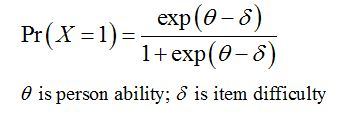The ability parameter and item difficulty parameter can be jointly estimated using joint maximum likelihood (JML) estimation method, for example. Under the marginal maximum likelihood (MML) estimation method, there is a further assumption about the ability distribution. In the simplest case, the ability distribution is assumed to follow a normal distribution with mean μ and variance σ2, as illustrated below: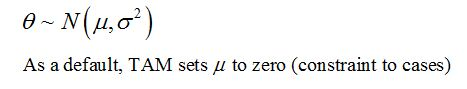Under MML, individual abilities θ are not estimated. Instead, the distribution parameters μ and σ2 are estimated. Individual ability estimates (e.g., WLE) can be computed after the model has been estimated. That's why in TAM, the tam.wle function applies AFTER the model has been estimated. Given this background information on MML, we now run the IRT analysis. Line 10 runs the MML estimation.
mod1 <- tam(scored)
The TAM function "summary" provides a summary of results (see line 11).
summary(mod1)
An excerpt of the summary output is shown below: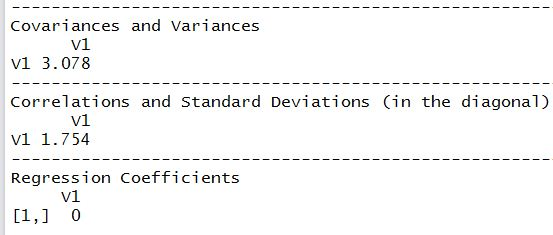The output shows that the estimated variance is 3.078 (sd=1.754), and the mean is 0 (see Regression Coefficient), as this is the default in TAM to set the mean of abilities to zero to define the origin of the ability scale.

Note that as σ2 is a parameter of the IRT model, it is "directly" estimated from the item response data. It is not estimated based on a two-step process where student abilities are first individually estimated, and then the variance of the estimated abilities is computed.

To illustrate this difference, a joint maximum likelihood (JML) estimation is run (see line 14), as JML makes no assumption about the population model.
mod0 <- tam.jml2(scored)
The variance of the abilities from the JML estimation can be obtained using
var(mod0\$WLE)
The variance is 2.56, somewhat different from the variance obtained using direct estimation (MML) (3.08). The under-estimation of the variance in the case of JML in this example is largely due to floor and ceiling effects (many students obtaining perfect and zero scores). In general, JML ability estimates tend to have larger variance than the variance estimated from MML because of measurement error contained in the individual ability estimates. The best way to explore the differences between different models is through simulation. The Extension section of this tutorial contains an example of simulation.

In summary, if you are interested in reporting population charateristics such as the mean and variance of the ability distribution, percentiles and histograms, etc., it will be better to use MML, since there is a population model assumption in computing the likelihood of item responses and the estimation of population characteristics is "direct" and not a two-step process. On the other hand, if you are only interested in providing individual student ability estimates and/or item estimates, then JML method is a better choice.

## Comparing two groups

In the data set for this tutorial, item responses for Taiwan and Australia are included. Suppose we are interested in comparing the ability distributions of these two countries. Before running an IRT analysis, we will first explore the raw data in a little more depth.

Lines 18 to 21 set up a "country" variable, where Australia (country code 36) is coded as country 1, and Taiwan (country code 158) is coded as country 2.
country <- resp[,12])
country[country==36] <- 1 # Australia
country[country==158] <- 2 # Taiwan
table(country))
There are 1050 Australian students and 719 Taiwan students in the data set.

### Compute raw scores

We will first take a look at the distribution of test scores for each country. Line 24 computes the raw score on the test for each student.
raw_score <- rowSums(scored)
As the item responses have already been scored, the sum of each row is the test score for a student.
Line 28 produces a cross-tabulation of the values of variables "country" and "raw_score". The following is the frequency table from this command: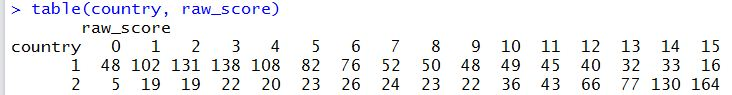It can be seen from this frequency table that the performances of the two countries differ greatly, with Taiwan students performing much higher on this test.
Lines 31-34 plot two histograms of test scores on the same graph in a new window.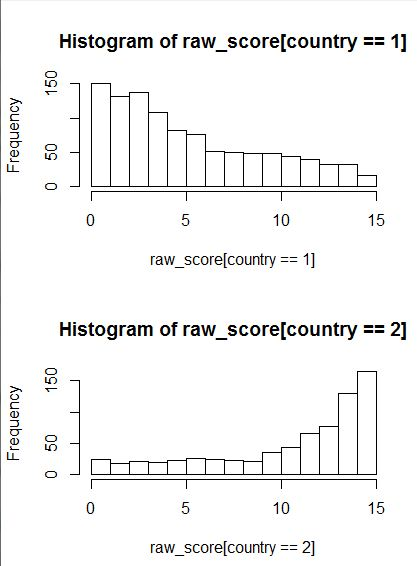As the histograms show, there is a considerable floor and ceiling effect if we use test raw scores to build population distributions of abilities. The use of MML estimation method with a population model assumption can address some of the problems caused by floor and ceiling effects.

For comparing two or more groups of students, the population model needs to take into account of different distributions for the groups. For example, for two groups of students, the population model may assume two separate normal distributions as illustrated below: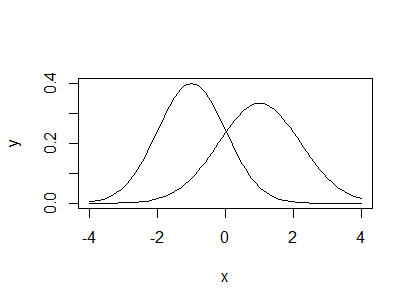In this way, the mean and variance for each group are explicitly modelled, and estimations of these parameters are "direct" in the sense that they are directly estimated from the item responses. In contrast, if we use the IRT model estimated in "mod1" (see line 10 of R script file), then only an overall mean and variance are estimated in the IRT run, and not the group means and variances. Parameters for groups will need to be estimated through the generation of ability estimates first. If model 1 is run (mod1 in the R script file) where the population model makes no distinction between the two groups, the model is actually "mis-specified" for any comparison of the two groups.

Line 41 of the R script file runs an IRT model where the population model assumes separate normal distributions for the two countries.
mod2 <- tam(scored, group=country)
Summary results from mod2 are shown below: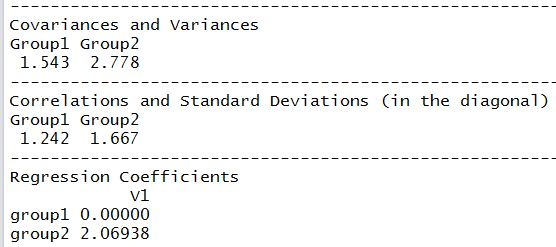The estimated variances for the two groups are 1.54 and 2.78 respectively, and the mean scores are 0 and 2.06 (under the heading of regression coefficients). The TAM default is to set the mean for group 1 to zero. The results show that not only the two countries differ in mean performance, but the variances are quite different too.

## Extension

In this section, a simulation study is presented to compare JML and MML in recovering population parameters. See R code from line 44 to line 63. Item responses are simulated to conform to the Rasch model. First, use JML to estimate item and person parameters. The mean and variance of the ability distribution is computed from WLE ability estimates. The estimated variance is larger than simulated variance (1.4 versus 1). This is a two-step process where the abilities of individuals are estimated, and then population characterisitcs are formed based on individual ability estimates.
In contrast, use the MML estimation method. The mean and variance of the ability distribution are parameters of the IRT model, so they are "directly" estimated. In this example, the estimated variance is 1.01. The mean is set to zero as this is the default constraint.
As an exercise, vary the sample size (N) and test length (I) to see how these affect the estimation of the mean and variance of the ability distribution uing JML and MML.For example, change I to 5 and 40.